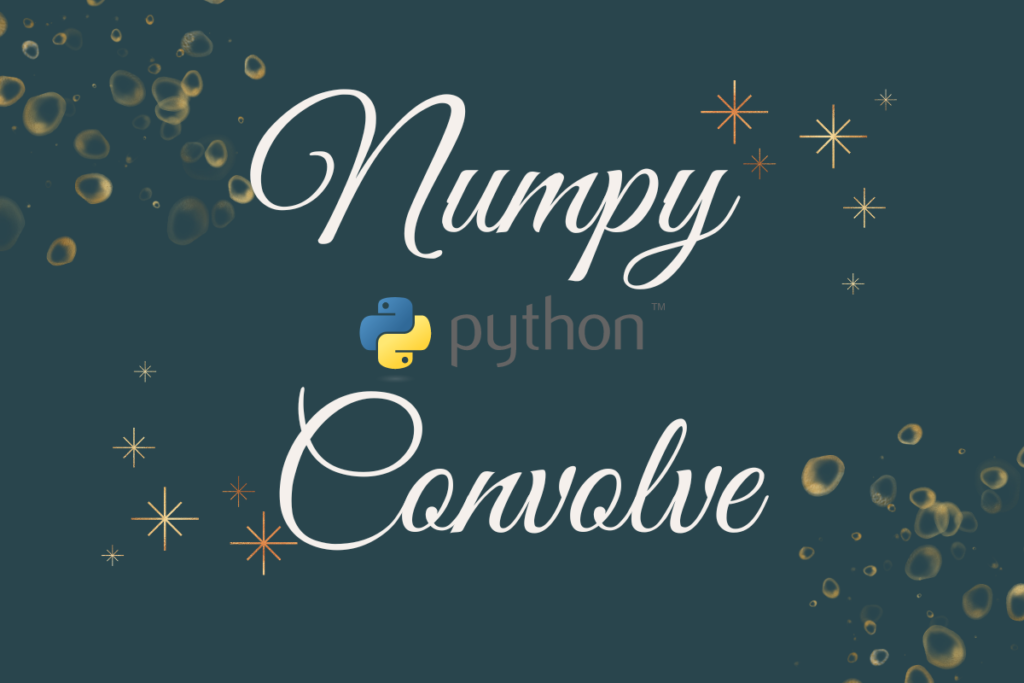# How to use Numpy Convolve in Python?Digital electronics always rely on processing signals for their routine functioning. The support from Python extends to this part of the spectrum too!

The operation of combining signals is known as convolution and Python has an exclusive function to carry it out. This function lies within the numpy library. So, let us start by importing it using the code below.

```import numpy as np
```

Thereafter, we shall explore further the convolve( ) function through each of the following sections.

• The convolve( ) function – explained
• Syntax of the convolve( ) function
• Use cases for the convolve( ) function

Also read: Numpy interp – One-dimensional linear interpolation for monotonically increasing sample points

## The convolve( ) function – explained

The mathematical technique by which two signals are combined together to form a third signal is known as convolution. The probability theory states that the sum of two independent random variables could be distributed only in accordance with the convolution of their individual distributions.

Convolution is the most critical know-how for someone who is into digital signal processing. The convolve( ) function from the numpy library deploys two distinct methods to carry out this technique.

• Linear convolution
• Discrete convolution

## Syntax of the convolve( ) function

Given below is the syntax of the convolve( ) function with its different components required for its functioning. It is to be noted that the function can be executed only if the inputs are given as one-dimensional arrays.

```numpy.convolve(a, v, mode=’full’)
```

where,

• a – First one-dimensional array of length N
• v – Second one-dimensional array of length M
• mode – An option set to full by default which is used to state the type of convolution that is to be carried out. It provides three categories to choose from viz.
• full – used to return the convolution at each point of overlap. The shape of the output is given by (N+M-1)
• same – used to return the output as given by max(M, N)
• valid – used to return the output as given by max(M, N) – min(M,N) + 1

It is to be noted that at full & same modes, the points on the signals do not overlap completely. But in the case of valid mode, the convolution product is only returned for the points where the signals overlap completely.

Also one has to bear in mind that if the array at position ‘v’ is longer than that at position ‘a’, then Python takes the privilege of swapping their positions before the code is run.

Also read: How to Use Numpy Positive in Python?

## Use cases for the convolve( ) function

Let us construct a pair of one-dimensional arrays. Once done, assign them to variables a & v respectively.

```a = np.array([1, -1, 9, 0])
v = np.array([5, 7, -6, 4])
```

Now let us deduce the convolution product with the default setting using the following code.

```np.convolve(a, v)
```

Let us now include the mode option for the same input arrays to change its default setting into the same mode using the code given below.

```np.convolve(a, v, mode = ‘same’)
```

Following is the output array returned.

```Output: array([  2,  32,  73, -58])
```

It is time to demonstrate the valid mode for the same input arrays by changing the above code as follows.

```np.convolve(a, v, mode = ‘valid’)
```

Following is the output array returned.

```Output: array()
```

One can observe the change in the length of the output when the same input arrays are run with each of the modes. This bears evidence to the logic of determining the length of the outputs as stated in the syntax section earlier.

Also read: How to find the QR Factorization of Matrix using Numpy?

## Conclusion

Now that we have reached the end of this article, hope it has elaborated on how to use the convolve( ) function from the numpy library. Here’s another article that details the usage of the linalg.qr( ) function from the numpy library in Python. There are numerous other enjoyable and equally informative articles in AskPython that might be of great help to those who are looking to level up in Python. Carpe diem!